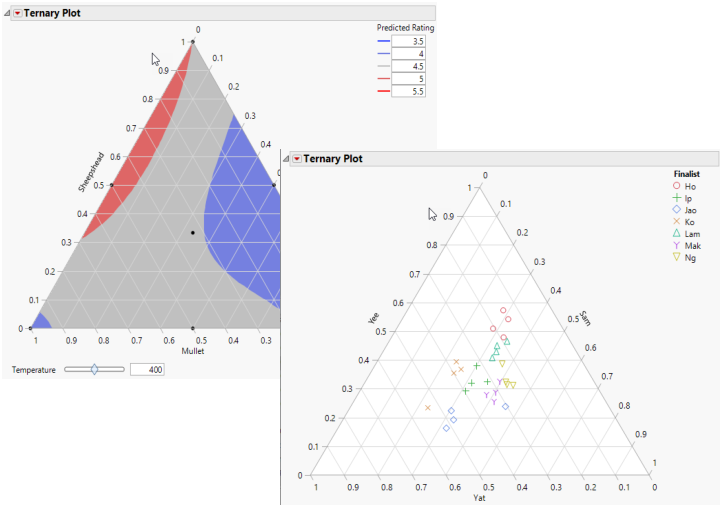Essential Graphing > Ternary Plots
Publication date: 11/29/2021

# Ternary Plots

##### View Plots for Compositional or Mixture Data

The Ternary Plot command in the Graph menu produces a three-axis plot.

Ternary plots are a way of displaying the distribution and variability of three-part compositional data. (For example, the proportion of sand, silt, and clay in soil or the proportion of three chemical agents in a trial drug.) You can use data expressed in proportions or use absolute measures.

The ternary display is a triangle with sides scaled from 0 to 1. Each side represents one of the three components. A point is plotted so that a line drawn perpendicular from the point to each leg of the triangle intersect at the component values of the point.

Figure 11.1 Examples of Ternary Plots